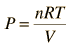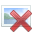# PV Work for Electrolysis Using Van Der Waals Equation

## Homework Statement

Solve for the work done during water electrolysis using the Van Der Waals equation.[/B]

Solving for work using Ideal Gas Law:This is the system work for the electrolysis of water using the Ideal Gas Law:

W = PΔV = (101.3 x 103 Pa)(1.5 moles)(22.4 x 10-3 m3/mol)(298K/273K) = 3715 J

http://hyperphysics.phy-astr.gsu.edu/hbase/thermo/electrol.html

## Homework Equations

[/B]
Van der Waals Equation## The Attempt at a Solution

[/B]
For 1 mL of water:

w= [nRT/(V-nb) − (an^2)/V^2] dV

a= 5.536 b=0.03049

w= [(1.5)( 8.3144621)(298)/(0.001)-(1.5)(0.03049)] − (5.536)(1.5)^2)/(0.001)^2] d(0.001)

w = -8739.4 J

Borek
Mentor
This is wrong on several levels, but it is hard to comment on not knowing what the real question is.

This is an example of solving for work done during water electrolysis using the Ideal Gas Law. I'm trying to solve for work using the Van Der Waals equation, but as you can see I'm having severe difficulty understanding how. Could you create a different scenario if necessary, just to show how the Van Der Waals equation could be used to solve for work done during electrolysis? I can't find any examples online.

Borek
Mentor
First problem is, you are sloppy with your math. You claim to be starting with 1 mL of water, yet you use 1,.5 moles in your calculations.

In general using Van Der Waals equation is not much more difficult than using just the ideal gas equation. Sure, more numbers to keep track of, but the logic behind the process is exactly the same, you calculate final volume and subtract initial.

First problem is, you are sloppy with your math. You claim to be starting with 1 mL of water, yet you use 1,.5 moles in your calculations.

In general using Van Der Waals equation is not much more difficult than using just the ideal gas equation. Sure, more numbers to keep track of, but the logic behind the process is exactly the same, you calculate final volume and subtract initial.

I apologize for the math. I'll use 18 mL next time. But what also confuses me is the volume change. Using the thread I posted earlier as an example, I think the volume is supposed to be constant and the temperature is supposed to change. I'm just not sure how to use T1 and T2 in my Van Der Waals equation.

Borek
Mentor
What T1 and T2? There is only one T in the VDW equation, and in the old thread you have stated that reaction takes place at 298 K.

I have no clue how to approach solving for work done during electrolysis using the Van Der Waals equation. I only plugged in the values I thought might work to at least attempt a solution. I started using the information from this thread which uses Ideal Gas Law to solve for work done:

This is the system work for the electrolysis of water using the Ideal Gas Law:

W = PΔV = (101.3 x 103 Pa)(1.5 moles)(22.4 x 10-3 m3/mol)(298K/273K) = 3715 J

In this thread, Dr. Claude explains that (298K/273K) is used because P1V1 = n1RT1 and P2V2 = n2RT2. Since V and n are constant, we are left with T2/T1 or 298K/273K. I only used 298K, because I didn't know how or even if T1 and T2 would be used in the Van Der Waals equation. I'm extremely confused. If you have any resources on how to use the Van Der Waals equation to solve for work done during electrolysis I'd be most grateful. I've been searching for weeks and haven't found much.

Here's the very first site I used when I first started learning about system work during electrolysis:

http://hyperphysics.phy-astr.gsu.edu/hbase/thermo/electrol.html

It contains more details regarding the W = PΔV equation used above. Thanks again for all of your help.

Borek
Mentor
W = PΔV

That is all that is needed to calculate work. Your problems stem from the fact you don't understand basics of the ideal gas behavior. Using two temperatures was a shortcut for calculating molar volume of the gas at 298 K from the known volume at 273 K - convenient when you know what you are doing, extremely confusing when you don't.

Best suggestion I have is to start from the very beginning - learn about ideal gas equation, do some problems involving converting numbers of moles to volumes, calculating new volumes/pressures/temperatures when other parameters change and so on. Things like 298/273*22.4 should automatically fall in place after that.

And don't worry about VDW equation, as I explained earlier - despite looking more complicated it is not that different.

Could you at least offer an example of using VDW to solve for work during electrolysis?